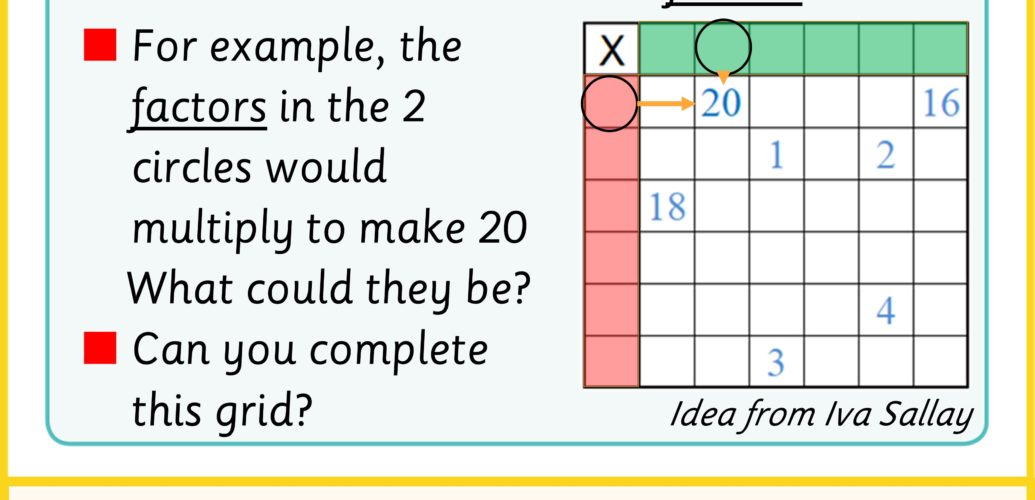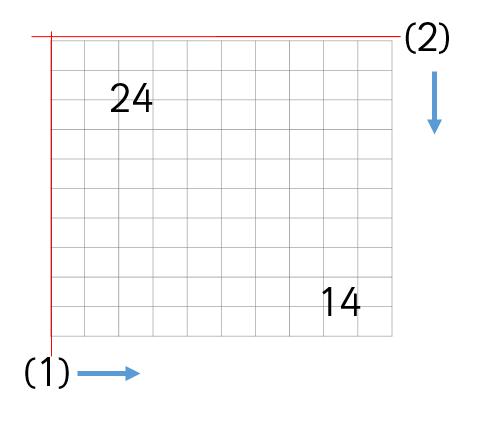# The X factors (and products)

This series of challenges are based on multiplication tables and mainly introduce the idea that you can create your own tables by choosing the factors and working out all the products.

### Challenge On Boarding – Multiplication Grids

#### Rows and Columns

We can organise sets of multiples in rows.  If we take all of the multiples of 1 and put them next to each other, and then all the multiples of 2 and so on, we end up with the table below. Each row is a times table.

We can see the 6 times table in the row that is coloured white.

It turns out that each column is also a times table (e.g. the 7 times table is the column in white)

This becomes a really useful way to organise multiplication facts.

It’s so useful and so well suited to multiplication that it is worth learning how to use it along side learning about multiplication.

To start with, practise times tables by isolating one row or column and read off the multiples.  Then cover and try to recall them.

### Challenge On Boarding – Factors and Products

A basic multiplication fact consists of factors (at least two) and a product (the result of the multiplication)

The multiplication grid organises all the possible multiplication facts for a range of numbers (in this case, 1 to 10)

Some factors can only be found in the grid twice.  Try colouring in those numbers that are only in this grid twice in red.

What about numbers that can be found 4 times?  Colour those in blue.

There are some numbers that can only be found once or 3 times.  These are special numbers. Colour them purple.

### Challenge On Boarding – 36 is the product. What are the factors?

If we have a product such as 36, there are only so many whole number factors that can produce it.  If we limit our factors to 1 – 6, there is only one multiplication that can work.

If we are allowed larger factors, there will be more possible ways to produce 36.

We have to use this idea to solve puzzles of this type.  You are given some products and you need to work out the factors

Can you work out two different ways to find factors that fit this table?

What about with a constraint that you must use each digit from 1 – 6 as a factor once?

### Challenge On Boarding – Using the area model

You can show these puzzle types using an area model.  For example, here is a puzzle in multiplication table format(1) How far along should the vertical line go? (2) How far down should the horizontal line go?

These are just 2 different representations for the same idea.  You can convert a puzzle like this:

### The Challenges

#### Challenge 1 – Find the factors

Check out the Find the factors website for lots more challenges of this type. They are created by Iva Sallay.

#### Challenge 3 – Can you arrange these products in a multiplication grid and find the right factors?

Download or share all of these challenges with this pdf and let us know how you got on with it by tweeting using #momentofmaths and start to see Every moment is a #momentofmaths.